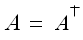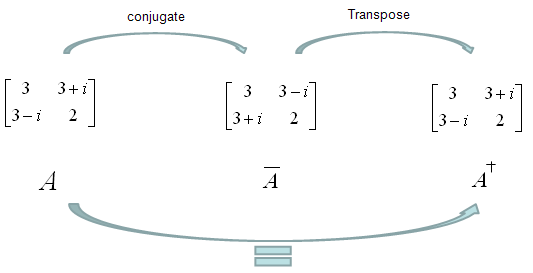Engineering Math - Matrix Hermitian Matrix   Hermitian Matrix is a special type of  matrix, which is same as its conjugate transpose as expressed below.In orther words, a Hermitian Matrix has following properties The entries on the main diagonal are real. The element in the ith row and jth column is equal to the complex conjugate of the element in the jth row and ith column Eigenvalues of all Hermitian Matrix are all real     One example of Hermintian Matrix is as follows. In this example, if you conjugate the matrix A and then transpose it, the result is same as the original matrix (A). So you can say the matrix 'A' is a Hermitian matrix.One of the important characteristics of Hermitian Matrix is that Eigenvalues of all Hermitian Matrix are all real as shown in the following example.Related Reading : Hermitian Conjugate, Conjugate Transpose